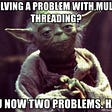# Problem

Alice and Bob each created one problem for HackerRank. A reviewer rates the two challenges, awarding points on a scale from 1 to 100 for three categories: problem clarity, originality, and difficulty.

The rating for Alice’s challenge is the triplet a = (a, a, a), and the rating for Bob’s challenge is the triplet b = (b, b, b).

The task is to find their comparison points by comparing a with b, a with b, and a with b.

• If a[i] > b[i], then Alice is awarded 1 point.
• If a[i] < b[i], then Bob is awarded 1 point.
• If a[i] = b[i], then neither person receives a point.

Comparison points is the total points a person earned.

Given a and b, determine their respective comparison points.

# Example

a = [1, 2, 3]
b = [3, 2, 1]

• For elements *0*, Bob is awarded a point because a .
• For the equal elements a and b, no points are earned.
• Finally, for elements 2, a > b so Alice receives a point.

The return array is [1, 1] with Alice’s score first and Bob’s second.

# Function Description

Complete the function compareTriplets in the editor below.

compareTriplets has the following parameter(s):

• int a: Alice’s challenge rating
• int b: Bob’s challenge rating

# Return

• int: Alice’s score is in the first position, and Bob’s score is in the second.

# Input Format

The first line contains 3 space-separated integers, a, a, and a, the respective values in triplet a.
The second line contains 3 space-separated integers, b, b, and b, the respective values in triplet b.

Constraints

• 1 ≤ a[i] ≤ 100
• 1 ≤ b[i] ≤ 100

Sample Input

`17 28 3099 16 8`

Sample Output

`2 1`

`fun compareTriplets(a: Array<Int>, b: Array<Int>): Array<Int> {var alicePoints = 0var bobPoints = 0   for (x in 0 until a.size) {      val itAlice = a[x]      val itBob = b[x]      if (itAlice > itBob) {         alicePoints++      } else if (itAlice < itBob) {        bobPoints++      }   }return arrayOf(alicePoints, bobPoints)}`

Explanation

in this example:

a = (a, a, a ) = (5,6,7)

b = (b, b, b) = (3,6,10)

Now, let’s compare each individual score:

a > b, so Alice receives 1 point.

a =b, so nobody receives a point.

a <b, so Bob receives 1 point.

Alice’s comparison score is 1, and Bob’s comparison score is 1. Thus, we return the array [1,1].

Comparing the 0 elements, 17 < 99 so Bob receives a point.
Comparing the 1 and 2 elements, 28 > 16 and 30 > 8 so Alice receives two points.
The return array is [2,1].

--

--

--

Hey curious person, I am Software Developer

Love podcasts or audiobooks? Learn on the go with our new app.

## Strava Metro — Scaling Aligned edges## What is a “C” library? What is it for?## Big O Notation## 3 Things Counseling Can Teach Software Developers: Part 1## Plan your projects quickly with Path## Umar Syaid Himawan

Hey curious person, I am Software Developer

## Ideas and Solutions for Advent of Code 2021 in Kotlin — Part 3/4## compileOnly and runtimeOnly, My experiment with them.## Is the FinTech world Falling in love with Java?## Java 102 : Executor Service in concurrent Package.